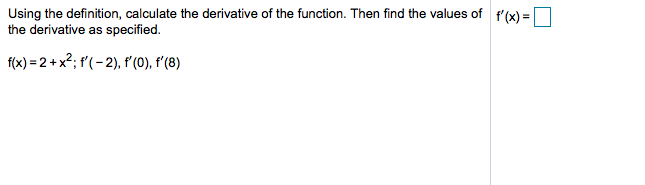# Using the definition, calculate the derivative of the function. Then find the values ofthe derivative as specified.f'(x)=f(x) 2+x2; (-2), f'(0), f'(8)

Question
1 viewshelp_outlineImage TranscriptioncloseUsing the definition, calculate the derivative of the function. Then find the values of the derivative as specified. f'(x)= f(x) 2+x2; (-2), f'(0), f'(8) fullscreen
check_circle

Step 1

The given function is f(x) = 2+x...

### Want to see the full answer?

See Solution

#### Want to see this answer and more?

Solutions are written by subject experts who are available 24/7. Questions are typically answered within 1 hour.*

See Solution
*Response times may vary by subject and question.
Tagged in

### Calculus